describe how light reflecting from a mirror can produce an image. In particular, explain how mirrors can produce images that are larger or

Question

describe how light reflecting from a mirror can produce an image. In particular, explain how mirrors can produce images that are larger or smaller than life size, as well as upright or inverted.

in progress 0
5 months 2021-09-03T06:43:02+00:00 1 Answers 0 views 0

A larger image is produced when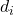>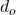A smaller image is produced when<An upright image  is produced when m is positive

An upright image  is produced when m is negative

Explanation:

The mirror equation is given as follows;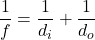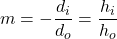For concave mirrors, f = focal length= Image distance from the mirror (-ve= Image is behind the mirror +ve= Image is in front of the mirror)= Object distance from the mirror (-ve= Object is behind the mirror +ve= Object is in front of the mirror)

m = Magnification (-ve m = Inverted image +ve m = upright image)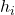= Image height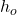= Object height

f = Focal length of the mirror

To produce a larger image>To produce a smaller image<To produce an upright image, m should be positive hence,will be negative or the image will appear behind the mirror

To produce an inverted image, m should be negative hence,will be positive or the image will form in front of the mirror.